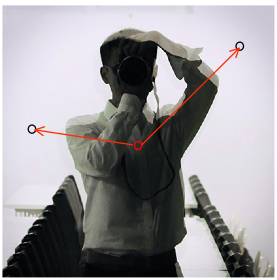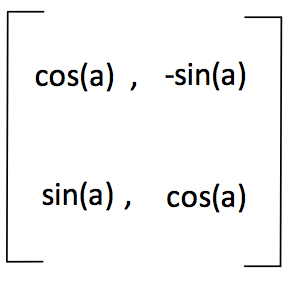# 【数字图像处理】MATLAB实现图像旋转

### 图像旋转原理

【ps：线性代数的本质这个视频很直观地解释了各种线性代数运算的实质，链接：https://www.bilibili.com/video/av6731067pp = R*(p-c) + c

### 代码实现过程

% 读入图片
im = imread('1.jpg');

% 求出旋转矩阵
a = 30 / 180 * pi;
R = [cos(a), -sin(a); sin(a), cos(a)];

% 求出图片大小 ch为通道数 h为高度 w为宽度
sz = size(im);
h = sz(1);
w = sz(2);
ch = sz(3);
c = [h; w] / 2;

% 初始化结果图像
im2 = uint8(zeros(h, w, 3));
for k = 1:ch
for i = 1:h
for j = 1:w
p = [i; j];
% round为四舍五入
pp = round(R*(p-c)+c);
if (pp(1) >= 1 && pp(1) <= h && pp(2) >= 1 && pp(2) <= w)
im2(pp(1), pp(2), k) = im(i, j, k);
end
end
end
end

% 显示图像
figure;
imshow(im2);

% 读入图片
im = imread('1.jpg');

% 求出旋转矩阵
a = 30 / 180 * pi;
R = [cos(a), -sin(a); sin(a), cos(a)];
R = R'; % 求出旋转矩阵的逆矩阵进行逆向查找

% 计算原图大小
sz = size(im);
h = sz(1);
w = sz(2);
ch = sz(3);
c1 = [h; w] / 2;

% 计算显示完整图像需要的画布大小
hh = floor(w*sin(a)+h*cos(a))+1;
ww = floor(w*cos(a)+h*sin(a))+1;
c2 = [hh; ww] / 2;

% 初始化目标画布
im2 = uint8(ones(hh, ww, 3)*128);
for k = 1:ch
for i = 1:hh
for j = 1:ww
p = [i; j];
pp = round(R*(p-c2)+c1);
% 逆向进行像素查找
if (pp(1) >= 1 && pp(1) <= h && pp(2) >= 1 && pp(2) <= w)
im2(i, j, k) = im(pp(1), pp(2), k);
end
end
end
end

% 显示图像
figure;
imshow(im2);

% 读入图片
im = imread('1.jpg');

% 求出旋转矩阵
a = 30 / 180 * pi;
R = [cos(a), -sin(a); sin(a), cos(a)];
R = R'; % 求出旋转矩阵的逆矩阵进行逆向查找

% 计算原图大小
sz = size(im);
h = sz(1);
w = sz(2);
ch = sz(3);
c1 = [h; w] / 2;

% 计算显示完整图像需要的画布大小
hh = floor(w*sin(a)+h*cos(a))+1;
ww = floor(w*cos(a)+h*sin(a))+1;
c2 = [hh; ww] / 2;

% 初始化目标画布
im2 = uint8(ones(hh, ww, 3)*128);
for k = 1:ch
for i = 1:hh
for j = 1:ww
p = [i; j];
pp = (R*(p-c2)+c1);
mn = floor(pp);
ab = pp - mn;
a = ab(1);
b = ab(2);
m = mn(1);
n = mn(2);
% 线性插值方法
if (pp(1) >= 2 && pp(1) <= h-1 && pp(2) >= 2 && pp(2) <= w-1)
im2(i, j, k) = (1-a)*(1-b)*im(m, n, k) + a*(1-b)*im(m+1, n, k)...
+ (1-a)*b*im(m, n, k)     + a*b*im(m, n, k);
end
end
end
end

% 显示图像
figure;
imshow(im2);

### 总结